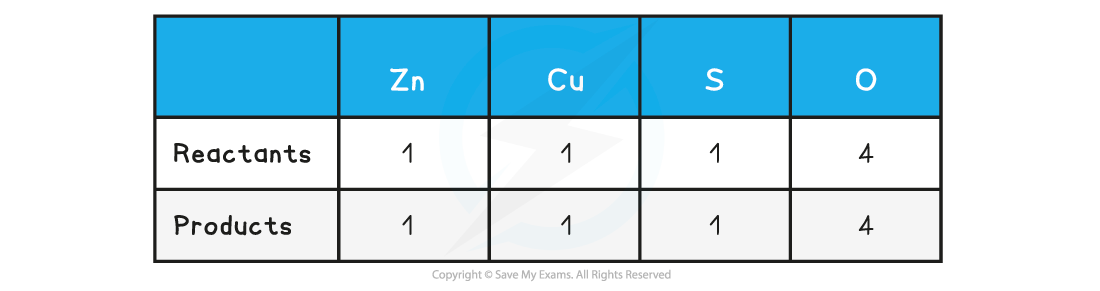# IB DP Chemistry: SL复习笔记1.1.2 Equations

### Balancing Equations

• A symbol equation is a shorthand way of describing a chemical reaction using chemical symbols to show the number and type of each atom in the reactants and products
• A word equation is a longer way of describing a chemical reaction using only words to show the reactants and products

#### Balancing equations

• During chemical reactions, atoms cannot be created or destroyed
• The number of each atom on each side of the reaction must therefore be the same
• E.g. the reaction needs to be balanced
• When balancing equations remember:
• Not to change any of the formulae
• To put the numbers used to balance the equation in front of the formulae
• To balance firstly the carbon, then the hydrogen and finally the oxygen in combustion reactions of organic compounds
• When balancing equations follow the following the steps:
• Write the formulae of the reactants and products
• Count the numbers of atoms in each reactant and product
• Balance the atoms one at a time until all the atoms are balanced
• Use appropriate state symbols in the equation
• The physical state of reactants and products in a chemical reaction is specified by using state symbols
• (s) solid
• (l) liquid
• (g) gas
• (aq) aqueous

#### Ionic equations

• In aqueous solutions ionic compounds dissociate into their ions
• Many chemical reactions in aqueous solutions involve ionic compounds, however only some of the ions in solution take part in the reactions
• The ions that do not take part in the reaction are called spectator ions
• An ionic equation shows only the ions or other particles taking part in a reaction, without showing the spectator ions

#### Worked Example

Balance the following equation:

Magnesium + Oxygen → Magnesium oxide

Step 1: Write out the symbol equation showing reactants and products

Mg + O2 → MgO

Step 2: Count the numbers of atoms in each reactant and productStep 3: Balance the atoms one at a time until all the atoms are balanced

2Mg + O2 → 2MgO

This is now showing that 2 moles of magnesium react with 1 mole of oxygen to form 2 moles of magnesium oxide

Step 4: Use appropriate state symbols in the fully balanced equation

2Mg(s) + O2(g) → 2MgO(s)

#### Worked Example

1. Balance the following equation

Zinc + copper(II) sulfate → Zinc sulfate + copper

2. Write down the ionic equation for the above reaction

Step 1: To balance the equation, write out the symbol equation showing reactants and products

Zn  + CuSO4  → ZnSO4 + Cu

Step 2: Count the numbers of atoms in each reactant and product. The equation is already balancedStep 3: Use appropriate state symbols in the equation

Zn (s)  + CuSO4 (aq)  → ZnSO4 (aq) + Cu (s)

Step 1:  The full chemical equation for the reaction is

Zn (s)  + CuSO4 (aq)  → ZnSO4 (aq) + Cu (s)

Step 2:  Break down reactants into their respective ions

Zn (s)  + Cu2+SO42- (aq)  → Zn2+SO42- (aq) + Cu (s)

Step 3:  Cancel the spectator ions on both sides to give the ionic equation

Zn (s)  + Cu2+SO42- (aq)  → Zn2+SO42- (aq) + Cu (s)

Zn (s)  + Cu2+(aq)  → Zn2+ (aq) + Cu (s)